HOME plateWON | World!OfNumbers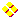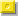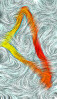Details of Palindromic Triangulars uptoThe Details  toThe Details  toFull ListingThe Subsets

Legend
```
F_ba  Decomposition of the basenumber in its prime factors.
F_pt  Decomposition of the palindromic triangular.
Note : a palindromic triangular will always be divisible by its basenumber when this
last one is 'odd'. With 'even' basenumbers you'll notice that the triangulars
are always divisible by half their basenumbers.
To reconstruct the triangular you must take into account the green together with
the purple prime factors but not the black 2.
Date  Date of discovery by the author.Hypertext links to related topics in number theory

```

Factorizations
```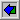F_ba  128184152897963669861552 =
2  x  2  x  2  x  2  x  11  x  17  x  127  x  1873  x  3581  x  50295245131
F_pt  8215588527084263951180440811593624807258855128 =
3  x  3243912979  x  13171762387769
Date  January 5, 2008 (Feng Yuan)F_ba  115945380367510626966648 =
2  x  2  x  2  x  3  x  193  x  8017  x  3122288398366517
F_pt  6721665614283359365218338125639533824165661276 =
7  x  11  x  131  x  1543  x  169633  x  43915229833
Date  January 5, 2008 (Feng Yuan)F_ba  114860741505066911342296 =
2  x  2  x  2  x  7  x  7  x  7  x  11  x  31  x  468151  x  262208746399
F_pt  6596494969546900318903773098130096459694946956 =
101  x  479  x  15420611  x  153961859113
Date  January 5, 2008 (Feng Yuan)F_ba  26539182748774333465497 =
3  x  3  x  3  x  677  x  19661  x  141101  x  523358663
F_pt  352164110486420593101030101395024684011461253 =
11  x  509  x  123553  x  19181995591667
Date  January 5, 2008 (Feng Yuan)F_ba  5805651147054009150041 =
1429  x  153395789  x  26485322761
F_pt  16852792620644766088388388066744602629725861 =
3  x  3  x  3  x  11  x  11  x  13  x  17  x  23  x  281  x  153469  x  4053449
Date  January 5, 2008 (Feng Yuan)F_ba  3630238456595636157728 =
2  x  2  x  2  x  2  x  2  x  113444951768613629929
F_pt  6589315625872933253747473523392785265139856 =
3  x  23  x  29  x  17234557  x  105265956197
Date  January 2, 2008 (Feng Yuan)F_ba  3261059106801402665754 =
2  x  3  x  3  x  3  x  3  x  3  x  23  x  23  x  149  x  4477841  x  19011299
F_pt  5317253249026181079052509701816209423527135 =
5  x  23  x  23  x  149  x  652211821360280533151
Date  January 2, 2008 (Feng Yuan)F_ba  775781766082836455602 =
2  x  11  x  337817  x  104384348772323
F_pt  300918674293302389819918983203392476819003 =
167  x  4645399796903212309
Date  May 31, 2001
Note the large palindromic substring in the largest prime factor
4645399796903212309F_ba  585863634063453017106 =
2  x  3  x  19  x  83  x  61917526322495563
F_pt  171618098859017793192291397710958890816171 =
11  x  53260330369404819737
Date  April 18, 2001F_ba  333741662509416495210 =
2  x  3  x  3  x  5  x  97  x  127  x  227  x  4523  x  293183831
F_pt  55691748647274629891519892647274684719655 =
17  x  37  x  530590878393348959
Date  April 18, 2000F_ba  333470345690102634085 =
5  x  23  x  21661  x  303959  x  440418821
F_pt  55601235727338276212021267283372753210655 =
100045471  x  1666593911533
Date  April 18, 2000
The first palindromic triangular discovered in this new millennium.

Add the triplets of the palindrome from right to left :
55 + 601 + 235 + 727 + 338 + 276 + 212 + 021 + 267 + 283 + 372 + 753 + 210 + 655 =  5005
... a nice palindromic number !F_ba  191600462227318472121 =
2  x  3  x  17  x  47  x  197  x  503  x  8693  x  92795011
F_pt  18355368562861046305450364016826586355381 =
2477  x  38675910825054193
Date  April 22, 2000F_ba  35369824079822102851 =
53  x  667355171317398167
F_pt  625512227718781732353237187817722215526 =
2  x  30253  x  8817409  x  33148469
Date  October 28, 1999 (after a two-year period of nonactivity...)F_ba  32637734114649927570 =
2  x  3  x  3  x  5  x  37  x  113  x  86735587219033
F_pt  532610844069291845737548192960448016235 =
7  x  11  x  47  x  193  x  241027  x  193869019
Date  December 30, 1997
Add the triplets of the basenumber from right to left :
32 + 637 + 734 + 114 + 649 + 927 + 570 =  3663
A nice palindromic number !

We perceive two palindromic substring of 4 digits in the basenumber.
326(3773)(4114)649927570
The difference between these numbers is 341.
This difference is a substring of the concatenation of these two palindromes :
37734114F_ba  19123354745855372721 =
3  x  17209  x  1199089  x  308912707
F_pt  182851348367914603505306419763843158281 =
9561677372927686361
Date  August 2, 1997
The following basenumbers start and end with the same digits :

 19 35755375665009 721
 19 123354745855372 721

A lot of palindromic subgroups can be extracted from this basenumber
19123354745855372721

19123354745855372721
19123354745855372721
19123354745855372721
19123354745855372721
19123354745855372721

19123354745855372721
19123354745855372721

Confert  the 'universal' number 42 is present :
Add the digits of the above basenumber together and divide by 2 !F_ba  16175904024612952346 =
2  x  3187  x  5347  x  474620309557
F_pt  130829935506744754616457447605539928031 =
3  x  7  x  13  x  746939  x  79326954001
Date  April 11, 1997
The Number of the Beast present as always in the base.
16175904024612952346

Extract the odd digits from the base and concatenate them to form this number
117591953. Now add the three triplets together. 117 + 591 + 953 =  1661
Yep, it's palindromic !

The digit 8 is the only one not showing up in the basenumber.

A triplet of palindromic duo's (77, 88 and 99)Sum up all the digits of the basenumber to arrive at a palindrome namely  77 .
Note that 77 x 88 =  6776  !The summation of the digits at either side of the middle number 1 of the palindromic
triangular number is the palindrome  88 .Add together the digits at the even places in the triangular number :
( 3 + 8 + 9 + 3 + 5 + 6 + 4 + 7 + 4 ) x 2 + 1 =  99

Let me play a little with the triplets of the triangular number.
The sum of the first two equals the sum of the last two triplets :
130 + 829     =     928 + 031     = the palindrome  959

Anagrams of the same numbers to be found in various places :
The sum of the first and the last three triplets of the triangular is
130 + 829 + 935 = 1894 and
1498 = 539 + 928 + 031
The fourth and fifth from left and right gives
506 + 744 = 1250 and
1052 = 447 + 605 and
1520 = 616 + 457 !
The third and fourth from left and right gives
935 + 506 = 1441 and
1144 = 605 + 539 !F_ba  11665833272979576316 =
2  x  2  x  9091  x  17761  x  18062451829
F_pt  68045832976478686977968687467923854086 =
11  x  844511  x  1255792165577
Date  January 29, 1997
The number 131 can be written as the sum of three consecutive primes
131  = 41 + 43 + 47F_ba  3654345456545434563 = PALINDROMIC
3  x  11  x  11  x  1429  x  9091  x  774923959
F_pt  6677120357887130286820317887530217766 =
2  x  17  x  101  x  149  x  849217  x  4205081
Date  May 17, 1996
Proof : this palindromic triangular is worth twice its basenumber.
Method : Divide into groups of three beginning from the right to the left :
Base = 003 + 654 + 345 + 456 + 545 + 434 + 563 = 3000
Tria = 006 + 677 + 120 + 357 + 887 + 130 + 286 + 820 + 317 + 887 + 530 + 217 + 766 = 6000

Odd and even are in perfect balance.
Method : split the odd and even numbers and add them together :
3 + 5 + 3 + 5 + 5 + 5 + 5 + 3 + 5 + 3   =   42
6 + 4 + 4 + 4 + 6 + 4 + 4 + 4 + 6     =   42
Now, let's apply this method with the palindromic triangular :
6 + 7 + 1 + 0 + 5 + 8 + 7 + 3 + 2 + 6 + 2 + 3 + 7 + 8 + 5 + 0 + 1 + 7 + 6 = 84 = 2 x 42
6 + 7 + 2 + 3 + 7 + 8 + 1 + 0 + 8 + 8 + 0 + 1 + 8 + 7 + 3 + 2 + 7 + 6   = 84 = 2 x 42
Again, the number 42 pops up !

The Number of the Beast present as always.
3654345456545434563

Note that the above sum 42 equals 6 * 6 + 6 and 6 equals 4 + 2

Each digit in the basenumber (except the border ones) is surrounded by two numbers
which sums up to either 8 or 10.
3
6 --> 3 + 5 = 8
5 --> 6 + 4 = 10
4 --> 5 + 3 = 8
3 --> 4 + 4 = 8
4 --> 3 + 5 = 8
5 --> 4 + 4 = 8
4 --> 5 + 5 = 10
5 --> 4 + 6 = 10
6 --> 5 + 5 = 10
5 --> 6 + 4 = 10
etc...

Clustered trios composed of odd digits emerge in this triangular number :
66 771 20 357 88 713 0286820 317 88 753 02 177 66
Assemble two numbers with those odd digits.
The first is 771357713 and the second is 317753177.
Performing adding and subtracting with these numbers yields interesting results :
771357713
- 317753177
---------------
= 453604536		note that 4536 occurs twice.
Remember that the digits 3, 4, 5 and 6 were the only 4 digits from our basenumber !

771357713
+ 317753177
---------------
= 1089110890		and 1089 also showing up twice.
Note that 4536 + its reversal 6354 equals 10890 which is also 1089 + its reversal 9801 !
Finally divide the whole addition number by two and we see  544555445  ... a palindrome !
This palindromic 'mean' value is a property we find with many numbers and their reversals.
It occurs when the sum of the number and its palindrome is even.
12345 + 54321 = 66666/2 = 33333
1089 + 9801 = 10890/2 = 5445
103 + 301 = 404/2 = 202F_ba  3652242206567626036 =
2  x  173  x  2957  x  1784851760869
F_pt  6669436567716980985890896177656349666 =
43  x  285451  x  297549720509
Date  May 16, 1996
This palindromic triangular is encapsulated by 'The Number of the Beast' itself
666 9436567716980985890896177656349 666
The sum of the digits of the middle part of the above triangular
equals the palindrome  181  !

A palindromic subgroup of 5 digits exists in the basenumber
3652242206567626036F_ba  3637582781740258588 =
2  x  2  x  19  x  37  x  3930491  x  329117339
F_pt  6616004247006598875788956007424006166 =
7  x  277  x  320741  x  5848986211
Date  May 16, 1996F_ba  3405603489389985674 =
2  x  7  x  43  x  467  x  29027  x  417328993
F_pt  5799067563472623134313262743657609975 =
3  x  5  x  5  x  11  x  4128004229563619
Date  April 30, 1996
Divide the basenumber in blocks of three from right to left and add them together :
003 + 405 + 603 + 489 + 389 + 985 + 674 = 3548
This is the basenumber of palindromic triangular !F_ba  3333229904907177885 =
3  x  3  x  5  x  29531  x  2508271838563
F_pt  5555210799483757064607573849970125555 =
13  x  109  x  761389  x  1544752211
Date  April 25, 1996F_ba  3271889143987456170 =
2  x  3  x  5  x  79  x  83  x  421  x  24109  x  1638743
F_pt  5352629285271484348434841725829262535 =
239  x  2023027  x  6767044007
Date  April 22, 1996F_ba  3237532871306389774 =
2  x  293  x  1483  x  1097909  x  3393197
F_pt  5240809546394698286828964936459080425 =
5  x  5  x  7  x  107  x  172898951738659
Date  April 20, 1996F_ba  2672899778869085022 =
2  x  3  x  83  x  241  x  499  x  727  x  61390523
F_pt  3572196613939201806081029393166912753 =
359  x  5941253  x  1253170549
Date  April 12, 1996F_ba  2470152923949718922 =
2  x  311  x  26293  x  151040465207
F_pt  3050827733848672937392768483377280503 =
3  x  73  x  571  x  19753480027427
Date  April 6, 1996F_ba  1935755375665009721 =
233  x  4721  x  1759788812897
F_pt  1873574437207991455541997027344753781 =
3  x  103  x  3132290251885129
Date  March 25, 1996A conjecture about the number 121 by Mike Keith.

The number 121 can be written as the sum of three consecutive primes
121  = 37 + 41 + 43F_ba  775527779120670322 =
2  x  1153  x  336308663972537
F_pt  300721668093919607706919390866127003 =
11  x  70502525374606393
Date  November 17, 1995F_ba  352465914177746748 =
2  x  2  x  3  x  3  x  3  x  271967  x  11999887043
F_pt  62116110328577368186377582301161126 =
107  x  413461  x  7967072987
Date  December 22, 1995F_ba  352449681516778876 =
2  x  2  x  19  x  30338993  x  152855957
F_pt  62110389000639430803493600098301126 =
43  x  8196504221320439
Date  December 22, 1995F_ba  247184344095525797 =
913687  x  270535034531
F_pt  30550049982967649594676928994005503 =
3  x  13  x  78367  x  40438322923
Date  March 29, 1992F_ba  112540786741633183 =
17  x  67  x  173  x  22277  x  25637957
F_pt  6332714340212879669782120434172336 =
2  x  2  x  2  x  2  x  11  x  81239  x  3935525353
Date  October 11, 1995F_ba  109113947229482909 =
7  x  41  x  47  x  843559  x  9589259
F_pt  5952926739999190550919999376292595 =
3  x  3  x  5  x  11  x  13  x  17  x  498715422229
Date  September 30, 1995F_ba  108100203429839929 =
7  x  11  x  17  x  43  x  367  x  6343  x  825007
F_pt  5842826990786388228836870996282485 =
5  x  10810020342983993
Date  September 27, 1995F_ba  78358935395500837 =
7  x  11  x  719  x  29501  x  47976899
F_pt  3070061378158136996318518731600703 =
13  x  17  x  89  x  769  x  4679  x  553601
Date  October 2, 1995F_ba  26555908318527497 =
7  x  13  x  3931  x  74236369457
F_pt  352608133311018969810113331806253 =
3  x  73  x  60629927667871
Date  April 24, 1992F_ba  10686132500837474 =
2  x  5351  x  165569  x  6030823
F_pt  57096713912727488472721931769075 =
3  x  5  x  5  x  11  x  181  x  14591  x  4904593
Date  October 13, 1993Test for divisibility by 111F_ba  4500990532341401 =
7  x  12163  x  219311  x  241051
F_pt  10129457886113466431168875492101 =
3  x  11  x  29  x  223  x  10545357391
Date  July 5, 1991F_ba  4062262265478592 =
2  x  2  x  2  x  2  x  2  x  2  x  7  x  365201  x  24828929
F_pt  8250987356765633365676537890528 =
11  x  13513  x  19853  x  1376567
Date  September 20, 1990
Arrange the numbers of this basenumber 004.062.262.265.478.592
in the form of a rectangle of 3 by 6
0 0 4
0 6 2	In the second row appears the Number of the beast 666 !
2 6 2
2 6 5
4 7 8
5 9 2

Another way of displaying also reveals the number of the beast in an unexpecting way.
Divide the basenumber in blocks of four : 4062.2622.6547.8592
and arrange these blocks in the form of a rhombus from the right downwards to the left.
4
2 0
6 6 6
8 5 2 2
5 4 2
9 7
2
Count the first three blocks of four together
4062 + 2622 + 6547
and a palindromic result appears namely :  13231

A large subsection of the basenumber is palindromic
40_6226226_5478592

The number 109 is a prime.
The next following prime is 113.
When added together we find a palindrome  222  which is a repdigit numberF_ba  3729035890325943 =
3  x  3  x  43  x  811  x  26951  x  440849
F_pt  6952854335669501059665334582596 =
2  x  2  x  11  x  4106521  x  10319053
Date  March 27, 1991
This is a posting I came across in sci.math by Robert E Sawyer answering
the following question of Ray Van Raamsdonk :
Does anyone know why the number 108 was so popular in China and India ?
In Buddhism, as in the Indian religion(s) that preceded it, 108 is a number that signifies
"completion", "perfection", etc... It is surely no coincidence that
108 = (1)*(2*2)*(3*3*3)
although I've never seen this fact mentioned anywhere. (Usually, the explanation somehow
relates 108 to mystical intuitions about the number 9.) Some claim that Sanskrit was at
one time spoken using exactly 2 * 54 basic phonetic elements, and regardless of whether
this is true, the numerological significance is seen in the belief that it was so.
(The same is true of the claim that there are or were originally exactly 108 Upanishads.)
Also, there are most commonly 108 beads on a Hindu or Buddhist mala (rosary).
According to an article in the Buddhist magazine _Tricycle_, the West is heir to this
numerology through the game of baseball, whose inventor had Buddhist inclinations and so
made the standard baseball with 108 stitches, as well as the 9 innings and 3 strikes, etc.
From Properties of the number 72 :
The Chinese astrology has 36 beneficial stars and 72 malevolent stars, their sumconstitutes the sacred number 108.F_ba  3249909720631025 =
5  x  5  x  47  x  2765880613303
F_pt  5280956596126015106216956590825 =
3  x  3  x  3  x  6991  x  41141  x  209249
Date  October 11, 1990F_ba  362785412063728 =
2  x  2  x  2  x  2  x  269  x  929  x  2699  x  33617
F_pt  65806627603124642130672660856 =
29  x  307  x  2341  x  17406523
Date  February 16, 1990F_ba  353520620692923 =
3  x  37  x  167  x  563  x  33874033
F_pt  62488414627554945572641488426 =
2  x  47  x  4637  x  405527029
Date  February 12, 1990F_ba  326825312860029 =
3  x  19  x  5733777418597
F_pt  53407392563028082036529370435 =
5  x  32682531286003
Date  January 31, 1990
The number 949 pops up at the start and end of basenumber 
326.***.***.***.029
326 + 623 =  949  = 029 + 920

Consider the following basenumbers
 ***.***.***.860.029
 173.008.***.***.***

Now 173 + 029 = 202 and 008 + 860 = 868
Both numbers are palindromic AND their difference is
868 - 202 = 666
again The Number of the Beast !F_ba  173008538941238 =
2  x  7  x  11  x  11  x  13  x  7856168329
F_pt  14965977273291019237277956941 =
3  x  3  x  349  x  21317  x  2583887
Date  January 16, 1990
Consider the following basenumber
 173.***.538.***.238
***.008.***.941.***
Now it happens that 173 + 538 + 238 =  949  and palindromic
but at the same time is  949  = 008 + 941 !
The same technique but applied to the first and last numbers of each block of three
produces a similar finding :
1*3.***.5*8.***.2*8	13 + 58 + 28 =  99
***.0*8.***.9*1.***	08 + 91 =  99 		Nice coincidence.F_ba  164402190438221 =
36319  x  4526616659
F_pt  13514040110442624401104041531 =
3  x  11  x  139  x  3121  x  5741893
Date  January 14, 1990
 164.***.190.***.221
***.402.***.438.***

Notice that 164 + 190 + 221 =  575  is palindromic.F_ba  109237730189290 =
2  x  5  x  1503113  x  7267433
F_pt  5966440848454114548480446695 =
11  x  19  x  73  x  389  x  1409  x  13063
The prime number 101 can be written as the sum of five consecutive primes
101  = 13 + 17 + 19 + 23 + 29F_ba  50364608806281 =
3  x  7  x  11  x  37  x  103  x  57210341
F_pt  1268296910104884010196928621 =
349  x  12899  x  5593891
A palindromic subgroup of 6 digits exists in the basenumber
50364608806281F_ba  41801907377572 =
2  x  2  x  83  x  22769  x  5529859
F_pt  873699730201575102037996378 =
7  x  31  x  89  x  223  x  617  x  15731

```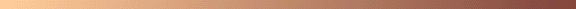[TOP OF PAGE]

Patrick De Geest - Belgium- Short Bio - Some Pictures